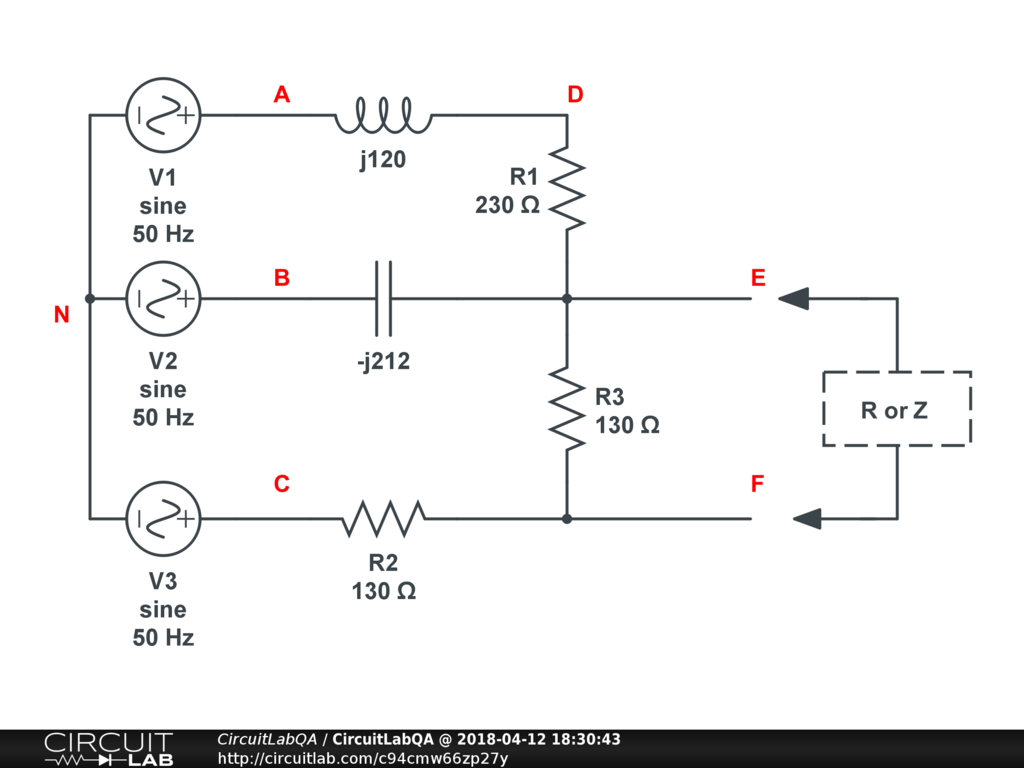## Help with circuit Millman/Thevenin/NortonThat's the circuit where the given values are: V(AN) = 150 /_10° [Vef] V(BN) = 150 /_-110° [Vef] V(CN) = 150 /_130° [Vef] Inductance is worth j120 [ohm] Capcacitor is worth -j212 [ohm] And the frecuency of the circuit is 50 [Hz] 1) I'm asked to find V(EN) with millman theorem and V(EN) with superposition theorem. 2) Thevenin and Norton values seen from nodes E and F. 3) The resistance that connected between E and F dissipates the optimum power and its value. 4) The impedance that connected between E and F dissipaste the maximum power and its value. by maxtraba April 12, 2018 Let me get this right - you want us to do your homework for you so that you don't learn anything and then fail your exams? by mikerogerswsm April 12, 2018

You must log in or create an account (free!) to answer a question.

### Ask a Question

Anyone can ask a question.

Did you already search (see above) to see if a similar question has already been answered? If you can't find the answer, you may ask a question.

CircuitLab's Q&A site is a FREE questions and answers forum for electronics and electrical engineering students, hobbyists, and professionals.

We encourage you to use our built-in schematic & simulation software to add more detail to your questions and answers.

Acceptable Questions:

• Concept or theory questions
• Practical engineering questions
• “Homework” questions
• Software/hardware intersection
• Best practices
• Design choices & component selection
• Troubleshooting

Unacceptable Questions:

• Non-English language content
• Non-question discussion
• Non-electronics questions
• Vendor-specific topics
• Pure software questions
• CircuitLab software support

Please respect that there are both seasoned experts and total newbies here: please be nice, be constructive, and be specific!